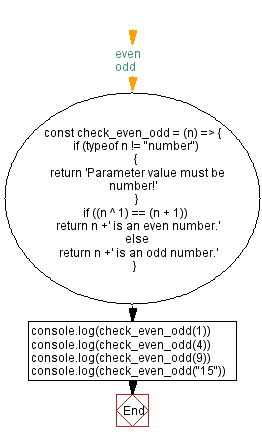# JavaScript - Odd or even number using bit manipulation

## JavaScript Bit Manipulation: Exercise-5 with Solution

Write a JavaScript program to check if a given number is odd or even using bit manipulation.

A number (i.e., integer) expressed in the decimal numeral system is even or odd according to whether its last digit is even or odd. That is, if the last digit is 1, 3, 5, 7, or 9, then it is odd; otherwise it is even-as the last digit of any even number is 0, 2, 4, 6, or 8.

Test Data:
(1) -> 1 is an odd number.
(4) -> 4 is an even number.
(9) -> 9 is an odd number.
("15") -> Parameter value must be number!

Sample Solution:

JavaScript Code:

``````const check_even_odd = (n) => {
if (typeof n != "number")
{
return 'Parameter value must be number!'
}
if ((n ^ 1) == (n + 1))//even
return n +' is an even number.'
else //odd
return n +' is an odd number.'
}
console.log(check_even_odd(1))
console.log(check_even_odd(4))
console.log(check_even_odd(9))
console.log(check_even_odd("15"))
```
```

Sample Output:

```1 is an odd number.
4 is an even number.
9 is an odd number.
Parameter value must be number!
```

Flowchart:Live Demo:

See the Pen javascript-bit-manipulation-exercise-5 by w3resource (@w3resource) on CodePen.

* To run the code mouse over on Result panel and click on 'RERUN' button.*

Improve this sample solution and post your code through Disqus

What is the difficulty level of this exercise?

Test your Programming skills with w3resource's quiz.

﻿